Practice the AP 9th Class Physical Science Bits with Answers Chapter 2 Laws of Motion on a regular basis so that you can attempt exams with utmost confidence.

## AP State Syllabus 9th Class Physical Science Bits 2nd Lesson Laws of Motion with Answers

Question 1.
The tendency of a body to resist change of state is
A) force
B) momentum
C) inertia
D) conservation
C) inertia

Question 2.
Law of inertia
A) Newton’s first law of motion
B) Newton’s second law of motion
C) Newton’s third law of motion
D) Law of conservation of mass
A) Newton’s first law of motionQuestion 3.
When the net force acting on a body is zero, the body is in
A) motion
B) rest
C) equilibrium
D) none
C) equilibrium

Question 4.
Mass of an object is considered as the measure of
A) conservation of mass
B) force
C) momentum
D) inertia
D) inertia

Question 5.
A non-zero net force acting on a body disturbs the state of
A) equilibrium
B) motion
C) rest
D) none
A) equilibrium

Question 6.
Effect of action of non zero net force on an object is explained by
A) Newton’s first law of motion
B) Newton’s second law of motion
C) Newton’s third law of motion
D) Law of conservation of mass
B) Newton’s second law of motion

Question 7.
Product of mass and velocity of an object is known as its
A) equilibrium
B) momentum
C) inertia
D) force
B) momentum

Question 8.
Momentum is a …………………. quantity.
A) scalar
B) vector
C) linear
D) constant
B) vector

Question 9.
Direction of momentum is in the direction of
A) mass
B) force
C) velocity
D) motion
C) velocityQuestion 10.
Acceleration is directly proportional to
A) mass
B) velocity
C) momentum
D) force
D) force

Question 11.
Acceleration is inversely proportional to
A) mass
B) velocity
C) momentum
D) force
A) mass

Question 12.
Net force is proportional to rate of change of momentum. This is
A) Newton’s first law of motion
B) Newton’s second law of motion
C) Newton’s third law of motion
D) Law of conservation of momentum
B) Newton’s second law of motion

Question 13.
1 kg. m/s2 =
A) 1 Dyne
B) 1 Hertz
C) 1 Newton
D) 1 Volt
C) 1 Newton

Question 14.
The effect of force exerted by one body on another body is explained by
A) Newton’s first law of motion
B) Newton’s second law of motion
C) Newton’s third law of motion
D) Law of conservation of mass
C) Newton’s third law of motion

Question 15.
The product of net force and interaction time is called
A) momentum
B) acceleration
C) conservation
D) impulse
D) impulse

Question 16.
Change in momentum depends on
A) magnitude of force
B) time
C) both A & B
D) none
C) both A & BQuestion 17.
When a net force acting on a system is zero, then the system is said to be
A) isolated system
B) system of mass
C) system of conservation
D) system of inertia
A) isolated system

Question 18.
Newton laws of motion are …………………
A) I law
B) II law
C) III law
D) All of these
D) All of these

Question 19.
The tendency of an object to resist a change in their state of rest or uniform motion is …………………..
A) rigidity
B) fluidity
C) inertia
D) compression
C) inertia

Question 20.
Newton’s first law of motion is also known as ……………………..
A) Law of friction
B) Law of force
C) Law of inertia
D) Law of momentum
C) Law of inertia

Question 21.
When the net acting on a body is zero, the body will be in ………………………
A) motion B) rest
C) acceleration
D) both A & B
D) both A & B

Question 22
………………… of an object is considered as the measure of inertia.
A) Volume
B) Pressure
C) Density
D) Mass
D) Mass

Question 23.
S.I unit of mass is ………………….
A) kg
B) gram
C) Newton
D) milligram
A) kgQuestion 24.
Mass is a property of an object that specifies how much …………….. the object has.
A) rigidity
B) fluidity
C) inertia
D) expansion
C) inertia

Question 25.
A non-zero net force acting on a body disturbs the state of ………………….
A) rest
B) motion
C) both
D) neither A nor B
C) both

Question 26.
Newton used the word ……………………. to represent momentum.
A) mass in rest
B) mass in stationary
C) mass in motion
D) none of these
C) mass in motion

Question 27.
Direction of momentum is direction of …………………
A) speed
B) velocity
C) acceleration
D) force
B) velocity

Question 28.
S.I unit of momentum is …………………..
A) kg.m/s2
B) kg-m/s
C) N.Sec
D) Both B & C
D) Both B & C

Question 29.
Acceleration increases with increase of …………………….
A) mass
B) pressure
C) volume
D) net force
D) net force

Question 30.
Acceleration decreases with increases of …………………..
A) mass
B) volume
C) density
D) pressure
A) massQuestion 31.
The unit of force is ……………
A) Newton
B) N.S
C) N/s
D) N.m
A) Newton

Question 32.
Force F = ……………………
A) ma
B) m($$\frac{\mathrm{v}-\mathrm{u}}{\mathrm{t}}$$)
C) $$\frac{\mathrm{mv}}{\mathrm{t}}$$
D) Both A & B
D) Both A & B

Question 33.
When an object exerts a force on other object, the result will be explained by ……………………… of motion.
A) Newton’s first law
B) Newton’s second law
C) Newton’s third law
D) None of these
C) Newton’s third law

Question 34.
In Newton’s third law of motion, the two opposing forces are known as …………………
A) reactant, product pair
B) action, reaction pair
C) force, retardation pair
D) none of these
B) action, reaction pair

Question 35.
When a net force acting on a system is zero, then that system is called …………………….
A) isothermal
C) isolated
D) isochoric
C) isolated

Question 36.
The product of net force and interaction time is called …………………
A) momentum
B) force
C) acceleration
D) impulse
D) impulse

Question 37.
The change in momentum that an object experiences during an interaction is equivalent to ………………….
A) momentum
B) couple
C) impulse
D) torque
C) impulseQuestion 38.
Change of momentum is related to ………………….
A) First law of motion
B) Second law of motion
C) Third law of motion
D) None of these
B) Second law of motion

Question 39.
The equation for law of conservation momentum is …………………
A) m1u1 – m2u2 = m1v1 – m2v2
B) m2u2 – m1u1 = m2v2 – m1v1
C) m1u1 + m2u2 = m1v1 + m2v2
D) $$\frac{m_{1} u_{1}}{m_{1} u_{2}}+\frac{m_{2} v_{2}}{m_{1} v_{1}}$$ = 0
C) m1u1 + m2u2 = m1v1 + m2v2

Question 40.
F net Δt is formula of …………………
A) acceleration
B) force
C) impulse
D) momentum
C) impulse

Question 41.
Momentum is given by ………………
A) $$\frac{\Delta \mathrm{s}}{\Delta \mathrm{t}}$$
B) $$\frac{\Delta \mathrm{v}}{\Delta \mathrm{t}}$$
C) mv
D) ma
C) mv

Question 42.
$$\frac{\Delta \mathrm{v}}{\Delta \mathrm{t}}$$is ……………….
A) force
B) momentum
C) displacement
D) acceleration
D) acceleration

Question 43.
Effect of action of no net force acting on an object
A) First law of motion
B) Second law of motion
C) Third law of motion
D) None of these
A) First law of motion

Question 44.
Effect of action of non-zero net force acting on an object
A) First law of motion
B) Second law of motion
C) Third law of motion
D) None of these
B) Second law of motionQuestion 45.
Assertion (A) : When a ball throw on the ground, it come to rest.
Reason (R) : Every object will remain rest when no net force acts on it.
A) A and R are true, R is the correct explanation of A.
B) A and R are true, R is not the correct explanation of A.
C) A is true and R is false.
D) A is false and R is true.
B) A and R are true, R is not the correct explanation of A.

Question 46.
Which of the following is wrong ?
a) Static inertia : The body at rest always tries to stay at the same rest, until some force act on it.
b) Dynamic inertia : The body which is in motion always tries to come to ? rest until some force act on it. ?
A) a
B) b
C) a and b
D) None
B) b

Question 47.
If there is no friction a moving ball
A) comes to rest
B) moves in uniform motion
C) moves with increasing speed
D) disappears
B) moves in uniform motion

Question 48.
We can push a bicycle easily, then a car because,
A) mass of the bicycle > mass of the car
B) mass of the car > mass of the bicycle
C) momentum of the car > momentum of the bicycle
D) momentum of the bicycle > momentum of the car
B) mass of the car > mass of the bicycle

Question 49.
A body is disturbed its state of equilibrium. Guess the reason.
A) A non – zero force acted on it
B) Zero net force acted on it
C) Either A or B
D) Neither A nor B
A) A non – zero force acted on it

Question 50.
Force : ma :: momentum :…………………….
A) m.f
B) mg
C) mv
D) 1/2mv2
C) mv

Question 51.
When we drop an egg on a soft pillow,
A) it do not break due to low impulse
B) it will break due to high impulse
C) A or B
D) it do not break due to high impulse
A) it do not break due to low impulseQuestion 52.
What will happen, if a non-net force acts on a body in a uniform motion ?
A) It accelerates
B) It decelerates
C) A or B
D) A and B
C) A or B

Question 53.
Formula a = b × c, says that the net force produces acceleration in a body in the direction of force, then the physical quantity of a, b and c are (respectively)
A) Fnet, mass, velocity
B) Fnet, mass, acceleration
C) Acceleration, mass, friction
D) mass, Fnet, gravitation
B) Fnet, mass, acceleration

Question 54.
Force : $$\frac{\mathbf{k g} \cdot \mathbf{m}}{\mathbf{s}^{2}}$$ :: ……… . : $$\frac{\mathbf{k g}-\mathbf{m}}{\mathbf{s}}$$
A) friction
B) acceleration
C) momentum
D) none
C) momentum

Question 55.What will happen in this experiment?
A) Cork cap and test tube moves in the same direction.
B) Cork cap and test tube moves in the opposite direction.
C) Tension in string decreases.
D) The cork cap drops in the test tube.
B) Cork cap and test tube moves in the opposite direction.

Question 56.
If a fly colloids with the wind sheild of a fast moving bus, then
a) the impact forces experienced by the fly and bus are same.
b) the acceleration experienced by the fly and bus are same.
A) ‘a’ only
B) ‘b’ only
C) both ‘a’ and b’
D) neither ‘a’ nor ‘b’
A) ‘a’ only

Question 57.
If a bird dashes a flying aeroplane,
A) bird will hurt seriously
B) aeroplane will damage
C) aeroplane will stop
D) A and B
D) A and BQuestion 58.
When a marble rolls down a slope, it picks up speed due to ……………….
A) normal force
B) frictional force
C) tension
D) force of gravity
D) force of gravity

Question 59.
When an object rolls up an inclined plane its speed ……………………
A)increases
B) decreases
C) no change
D) none of these
B) decreases

Question 60.
Materials required to show static friction in your lab are
A) bottle, paper, scale
B) glass, wooden planck, stand
C) bottle, paper, pen cap
D) test tube, cork, water
C) bottle, paper, pen cap

Question 61.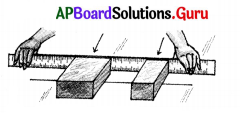Conclusion of the given experiment is
A) inertia of an object depends upon its mass.
B) inertia of an object depends upon its shape.
C) there is no relation between mass and inertia.
D) none of the above.
A) inertia of an object depends upon its mass.

Question 62.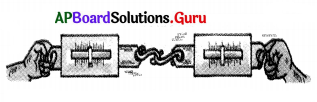This experiment is used to prove
A) Newton’s first law of motion
B) Newton’s second law of motion
C) Newton’s third law of motion
D) Newton’s Gravitational theory
C) Newton’s third law of motion

Question 63.
From the above given figure, your observation is, if we pull one of the spring balances, the reading in other spring balance
A) increases
B) decreases
C) no change
D) we cannot say
A) increases

Question 64.
To prove Newton’s third law of motions the required apparatus are
A) two weights
B) two test tubes
C) two scales
D) two spring balances
D) two spring balancesQuestion 65.
“The natural state of an earthly object is to be at rest.” This idea was concluded by
A) Galileo
B) Aristotle
C) Newton
D) Einstein
B) Aristotle

Question 66.
The statement: “An object in motion will remain in same motion as long as no external force is applied on it” is given by
A) Galileo
B) Aristotle
C) Newton
D) Einstein
A) Galileo

Question 67.
Laws of motion were proposed by
A) Galileo
B) Aristotle
C) Newton
D) Einstein
C) Newton

Question 68.
The connection between the force and change in motion was explained by ………………..
A) Kepler’s laws
B) Newton’s laws of motion
D) None of these
B) Newton’s laws of motion

Question 69.
For a body Fnet = 0
From the given data the velocity of the body is
A) zero
B) constant
C) A or B
D)A and B
C) A or B

Question 70.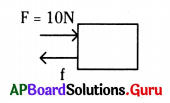The body is moving is steadily. Then the friction is
A) zero
B) 10 N
C) 10 × 9.8 N
D) none
B) 10 N

Question 71.
Tension in the string of an atwood machine is $$\frac{2 \mathrm{~m}_{1} \mathrm{~m}_{2} \mathrm{~g}}{\mathrm{~m}_{1}+\mathrm{m}_{2}}$$ and m1 = m2
From the given data tension in string equals to
A) weight
B) mass
C) gravitation
D) weight/2
A) weightQuestion 72.
FAB = – FBA From the data the following statement is false.
B) FAB is a force of a action
C) No single isolated force exists
D) It is an application of Newton’s third law

Question 73.
Below figure was best example for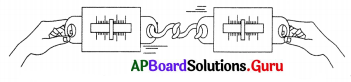A) Newtons first law of motion
B) Newtons second law of motion
C) Newtons third law of motion
D) None of these
C) Newtons third law of motion

Question 74.
Name the used system in the given figure.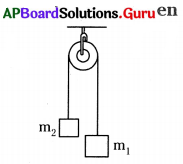A) At wood machine
B) Chain system
C) Momentum
D) None
A) At wood machine

Question 75.
Use of above system was
A) To prove the Newtons laws of motion
B) To find acceleration
C) Both A and B
D) None of the above
A) To prove the Newtons laws of motion

Question 76.
The free body diagram that shows forces acting on a book on a table is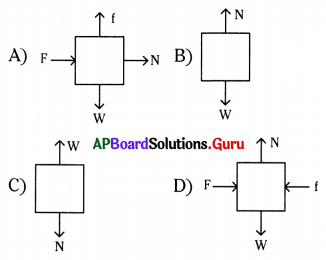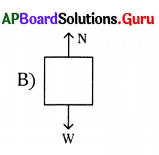Question 77.
A body of mass’m’ is kept on a table and it is pushed in the horizontal direction with force of 10N continuously. Free body diagram of the body is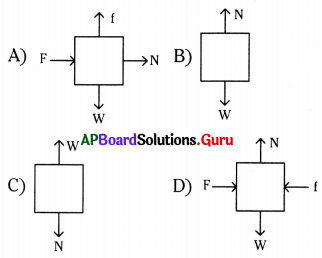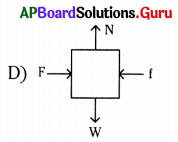Question 78.
Free body diagram of the given situation. “A monkey is hanging branch of a tree.”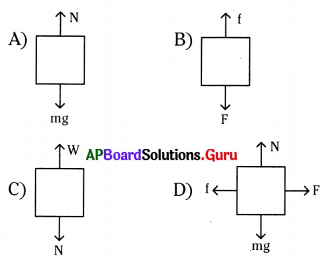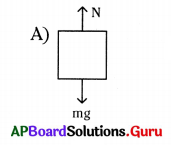Question 79.
The velocity of a small object that has separated from a rocket moving in free space with velocity of 11 km/s is
A) 0 km/s
B) 11 km/s
C) 11 × 9.8 km/s
D) None
B) 11 km/s

Question 80.
You are travelling in a bus with 40 km/hr speed. Then your speed related to the observer standing out side of the bus is
A) zero
B) 40 km/hr
C) 40 × 9.8 km/hr
D) none
B) 40 km/hr

Question 81.
A heavy truck and bicycle hit a wall, with same velocity. More damage to the wall expected from
A) heavy truck
B) bicycle
C) both
D) nothing will happen
A) heavy truck

Question 82.
If a badminton ball and a cricket ball hit you with same speed, which one hurts you more and why ?
A) Badminton ball – more momentum
B) Cricket ball – less momentum
C) Badminton ball – less momentum
D) Cricket ball – more momentum
D) Cricket ball – more momentum

Question 83.
The great phrase “mass in motion” instead of momentum is used by
A) Galelio
B) Aristotle
C) Newton
D) Einstein
C) Newton

Question 84.
m1 = 6.2 kg, m2 = 3.6 kg then tension in the string is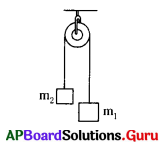A) 44. 64 N
B) 63.24 N
C) 22.32 N
D) None
A) 44. 64 N

Question 85.
Which of the following is not an application of Newton’s third law of motion ?
A) Flying of a bird
B) Swimming of a fish
D) None
D) NoneQuestion 86.
The force exerted by the earth on a ball is 8N. The force on the earth by the ball is
A) 8 × 9.8 N
B) 8N
C) 4N
D) Zero N
B) 8N

Question 87.
It is difficult for a fire fighter to hold a hose (pipe) that ejects large amount of water at high speed.
The Law involved in it is
A) Newton’s first law of motion
B) Newton’s 2nd law of motion
C) Newton’s 3rd law of motion
D) Newton’s 4th law of motion
C) Newton’s 3rd law of motion

Question 88.
The fielder pulls back his hands while catching a fast moving cricket ball. It gives ………………
a) less stopping distance
b) more stopping distance
c) less time to stop
d) more time to stop
A) a, c
B) b, d
C) a, d
D) b, d
B) b, d

Question 89.
The application of “impulse of force acts on the driver for longer time when accident occurs”.
A) Breaks of the vehicles
B) Air bags inside the vehicles
C) Breaking of the glass windows
D) Power stearing of the vehicle
B) Air bags inside the vehicles

Question 90.
Safety net to save a person who jumps from a height provides
A) low impulse
B) high impulse
C) low inertia
D) high inertia
A) low impulse

Question 91.
If you bite by a stick on your palm, how do you escape from a larger impulse on your hand ?
A) By pulling down your palm along the direction of the stick
B) By raising up your palm opposite to the direction of the stick
C) By giving no moment to your palm
D) By catch the stick with palm
A) By pulling down your palm along the direction of the stick

Question 92.
The following is not an application of the formule ΔP = FnetΔT (Here more Fnet)
A) egg drops on a concrete floor
B) hit by a bicycle
C) pulling hands back while catching a ball
D) jumping on a cement road
C) pulling hands back while catching a ballQuestion 93.
The following is not an application of the formula ΔP = Fnet ΔT
(Here more ΔT
A) egg dropping on a cushion
B) pulling hands back while catching a ball
C) opening of air balloons while hitting of a wall by a car
D) hitting of a ball to the body
D) hitting of a ball to the body

Question 94.
Principle involved in the parachutes
A) Longer time to hit the land-low impulse
B) Longer time to hit the land-high impulse
C) Shorter time to hit the land-low impulse
D) Shorter time to hit the land-high impulse
A) Longer time to hit the land-low impulse

Question 95.
When a carpet is beaten with a stick dust comes out due to
A) static inertia of dust
B) static inertia of carpet
C) dynamic inertia of dust
D) none
A) static inertia of dust

Question 96.
Luggage kept on the roof of a bus is tied with a rope, because it fall down due to
A) static inertia of the luggage
B) static inertia of the bus
C) both A and B
D) dynamic inertia of the luggage
A) static inertia of the luggage

Question 97.
Bowler In cricket runs in from a long distance before he bowls to provide
A) static inertia to the ball
B) dynamic inertia to the ball
C) both A and B
D) none
B) dynamic inertia to the ball

Question 98.
Which of the following has more inertia ?
A) 8 kg stone
B) 25 kg stone
C) 80 kg stone
D) All are equal
C) 80 kg stoneQuestion 99.
The momentum of a 6.0 kg bowling ball with a velocity of 3 m/s
A) 6 kg m/s2
B) 18 kg m/s2
C) 2 kg m/s2
D) 180 kg m/s2
B) 18 kg m/s2

Question 100.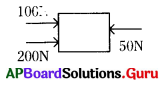What is the net force?
A) 350 N
B) 250 N
C) 50 N
D) None
B) 250 N

Question 101.
A passenger in moving train tosses a coin which falls behind him. It means that the motion of the train is
A) accelerated
B) uniform
C) retarded
D) circular motion
A) accelerated

Question 102.
If a car is travelling westwards with a constant speed of 20 m/s. The resultant force acting on it is
A) 20 m/s
B) 20 × 9.8 m/s
C) zero
D) 10 m/s
C) zero

Question 103.
A man of mass 30 kg uses a rope to climb which bears only 450 N. The maximum acceleration with which he can climb safely is
A) 45 m/s2
B) 30 m/s2
C) zero
D) 15 m/s2
D) 15 m/s2

Question 104.
A vehicle has a mass of 1500 kg. What must be the force between the vehicle and the road if the vehicle is to be stopped with a negative acceleration of 1.7 m/s2 ?
A) 2500 N in a direction opposite to that of the vehicle.
B) 2500 N in a direction of the vehicle.
C) 2550 N in a direction opposite to that of the vehicle.
D) 2550 N in a direction of the vehicle.
C) 2550 N in a direction opposite to that of the vehicle.

Question 105.
A truck is moving under a hopper with a constant speed of 20 m/s. Sand falls on the truck at a rate of 20 kg/s. The force acting on the truck due to falling of sand is
A) 40 N opposite to the motion of the truck
B) 400 N opposite to the motion of the truck
C) 40 N along the motion of the truck
D) 400 N along the motion of the truck
B) 400 N opposite to the motion of the truckQuestion 106.
A ball of mass 1 kg moves perpendicularly to a bat with a speed of 5 m/s. And the bat of 10 kg moves opposite to the ball with 2 m/s. After hitting ball moved with 15 m/s. What is the speed of bat after hit ?
A) 1 m/s
B) 2 m/s
C) 3 m/s
D) zero
A) 1 m/s

Question 107.
Identify the correct statement regarding Aristotle’s belief.
A) Any moving body naturally comes to rest.
B) Any body which is at rest naturally starts moving.
C) A body continues its state of rest or uniform motion unless a net force acts on it.
D) Any moving body continues in the state only until some external force acts on it.
A) Any moving body naturally comes to rest.

Question 108.
Match the physical quantities given in Set – A with proper units in Set – B.

 Set – A Set – B P. Force x. kg ms2 Q. Momentum y. kg ms-2 R. Impulse z. kg ms-1

A) P – x, Q – z, R – y
B) P – y, Q – z, R – x
C) P – z, Q – x, R – y
D) P – x, Q – y, R – z
B) P – y, Q – z, R – x

Question 109.
Pavithra : Action reaction pair in Newton’s third law always represents forces acting on two bodies simultaneously.
Teja : Newton’s first and second laws of motion apply to a single body.
A) Both Pavithra and Teja are incorrect.
B) Pavithra is correct, Teja is incorrect.
C) Pavithra is incorrect, Teja is correct.
D) Both Pavithra and Teja are correct.
D) Both Pavithra and Teja are correct.

Question 110.
When we drop an egg on the concrete floor it will break because
A) smaller force acts on the egg for shorter time.
B) smaller force acts on the egg for longer time.
C) large force acts on the egg for shorter time.
D) large force acts on the egg for longer time.
C) large force acts on the egg for shorter time.

Question 111.
When 2.5 kg weight of hero’s hand hits the villain of 80 kg who is at rest, the villain moves with 12.5 ms-1 and hits the transformer near by. What will be the speed of hero’s hand compared to the bullet fired from the gun ? (Speed of bullet fired from the gun is 370 ms-1)
A) Less than bullet speed
B) Very very less than bullet speed
C) 0
D) Greater than bullet speed
D) Greater than bullet speed

Question 112.
Assertion (A) : Pace bowler Bumrah runs in from a long distance before he bowls.
Reason (R) : To acquire dynamic inertia.
A) Both (A), (R) are correct, (R) is correct explanation of (A).
B) (A) is correct, (R) is incorrect.
C) (A) is incorrect, (R) is correct.
D) Both (A), (R) are correct, (R) is not correct explanation of (A).
B) (A) is correct, (R) is incorrect.

Question 113.
Take a block of ice and place it on a smooth surface, push the block with some net force. Now increase the net force and push it again. The outcome of the experiment is
A) Smaller the net force larger the acceleration
B) Smaller the net force smaller the acceleration
C) Larger the net force larger the acceleration
D) Larger the net force smaller the acceleration
C) Larger the net force larger the acceleration

Question 114.
Make a stack of carrom coins on the carrom board. Give a sharp hit at the bottom of the stack with the striker. The law which represent the above experiment is
A) Newton’s second law of motion
B) Newton’s third law of motion
C) Newton’s fourth law of motion
D) Newton’s first law of motion
D) Newton’s first law of motion

Question 115.
Read the passage given below :
Inertia is a property of matter that resists changes in its state of motion or rest. It depends on the mass of the object. Arrange the following animals in the increasing order of inertia :
a. Elephant
b. Lion
e. Dog
d. Rat
A) d, c, b, a
B) b, a, c, d
C) c, d, a, b
D) a, b, c, d
A) d, c, b, a

Question 116.Five pendulums are suspended as shown in figure, il first pendulum is pulled a side and released you will see that pendulum 5 will move a side, if you do it with first two, last two will move and so on.
What is the principle involved in the above activity?
A) Law of conservation of energy
B) Law of conservation of momentum
C) Law of conservation of charge
D) Law of conservation of mass
B) Law of conservation of momentum

Question 117.
Which of the following represent correct diagram of atwood machine?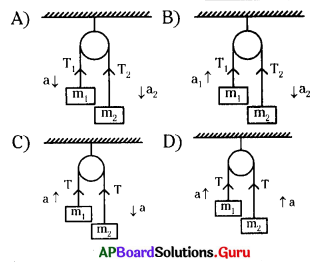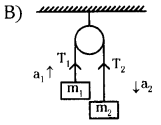Question 118.
A boy of mass 30 kg uses a rope to climb which bears nearly 600 N. What is the maximum acceleration with which he can climb safely ?
A) 0.05 ms-2
B) 20 ms-2
C) 0
D) 18000 ms-2
B) 20 ms-2

Question 119.
A ball of mass ‘m’ moves perpendicularly to a wall with a speed ‘v’ strikes it and rebounds with the same speed is the change in momentum.
A) $$\frac{1}{2}$$ mv
B) 2 mv
C) mv
D) 0
D) 0

Question 120.
The main apparatus in atwood machine
A) Pulley
C) Barometer
D) Spring balance
A) Pulley

Question 121.
Which of the following states the law of constant proportions ?
A) Matter is neither created nor destroyed during a chemicaPreaction.
B) A given chemical substance always contains the same elements combined in fixed proportion.
C) Atoms of different elements have different masses and chemical properties.
D) Compounds are formed when atoms of different elements combine in whole number ratios.
D) Compounds are formed when atoms of different elements combine in whole number ratios.

Question 122.
Which property of an object determines how much inertia it has ?
A) Acceleration of the object
B) Velocity of the object
C) Mass of the object
D) Shape of the object
C) Mass of the object

Question 123.
A force of IN acts on a body of mass 5kg. The body covers 100 cm in 2 seconds moving along a straight line. The initial velocity is
A) 200 cm/sec
B) 102 cm/sec
C) 0.5 cm/sec
D) 120 cm/sec
D) 120 cm/sec

Question 124.
Take two bricks of different masses and place them on a straight line on the floor. Push both with the same force at the same time with a wooden scale. Which of the following can be observed ?
A) The brick with greater mass moves the same distance as the brick with lesser mass.
B) The brick with greater mass moves more than the brick with lesser mass.
C) The brick with greater mass moves less than the movement of the brick with lesser mass.
D) The brick with greater mass will move and the brick with lesser mass will not move at all.
C) The brick with greater mass moves less than the movement of the brick with lesser mass.

Question 125.
When an object exerts a force on the other object, the second object also exerts a force on the first one which is equal in magnitude but opposite in direction.
Which of the following is BEST explained by the above ?
A) Newton’s first law of motion
B) Newton’s second law of motion
C) Newton’s third law of motion
D) Law of conservation of momentum
C) Newton’s third law of motion

Question 126.
NewtonSec is the unit for
A) Momentum
B) Inertia
C) Impulse
D) Force
A) Momentum

Question 127.
A suitcase kept in a moving bus moves forward when the bus.
A) Comes to rest
B) Moves forward
C) Takes turn
D) At rest
A) Comes to rest

Question 128.
Two people push a car for 2s with a combined net force of 250 N. The impulse providedjo the car is
A) 500 NS
B) 12$$\frac{\mathrm{N}}{\mathrm{S}}$$
C) 0
D) 250 N
A) 500 NS

Question 129.
In the paper ring activity of our text book we observe the result of
A) Force
B) Inertia
C) Velocity
D) Acceleration
B) Inertia

Question 130.
Which of the following law is illustrated by Balloon rocket activity :
A) Newton’s first law
B) Newton’s Second law
C) Newton’s Third law
D) Newton’s Law of Gravity
C) Newton’s Third law

Question 131.
S.I. Unit of Force is
i. kgms-2
ii. $$\frac{\mathrm{kg}}{\mathrm{ms}^{-2}}$$
iii. newton
A) iii only
B) ii and iii
C) i and iii
D) i, ii and iii